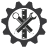SuperTeacherTools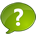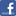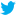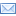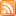Something not working? Try the new template: 6th Grade Math Jeopardy Jeopardy Review 6th Grade Math Jeopardy
&question25=

Find the circumference of a circle with a radius of 5 in.

C = 2∏ r

The Answer is:  126

The answer is:  83

The answer is 69

The answer is:  45

The student did not subtract 12 - 3 before squaring.  It should look like this:

2 × (12-3)2 + 7

2 × 92 + 7

2 × 81 + 7

162 + 7

169

The answer is:  35.4

The answer is:  2.940

7.5

61.1

1/2

4 4/5

6 39/60

16/35

7/40

1,2,4,8,16,32,64

16, 32, 48, 64, 80

84 - 1,2,3,4,6,7,12,14,21,28,42,84

120 - 1,2,3,4,5,6,8,10,12,15,20,24,30,40,60,120

GCF is 12

5- 5,10,15,20,25,30,35,40,45,50

6, - 6,12,18,24,30,36,42,48

10 - 10,20,30,40,50

LCM is 30

Look for the GCF

18 - 1,2,3,6,9,18

24 - 1,2,3,4,6,8,12,24

The GCF is 6.  Therefore the greatest number of students that can be in a row is 6.

Area is 180 yd2

7.5 in22

A = 337.5 cm

&value1=100&value2=200&value3=300&value4=400&value5=500&value6=100&value7=200&value8=300&value9=400&value10=500&value11=100&value12=200&value13=300&value14=400&value15=500&value16=100&value17=200&value18=300&value19=400&value20=500&value21=100&value22=200&value23=300&value24=400&value25=500&finalquestion=

Piano classes start at 9 am and are given every 60 minutes.  Violin classes also start at 9 am and are given every 45 minutes.  What is the next time at which a piano lesson and a violin lesson will start at the same time?

12 noon

&question25=

Find the circumference of a circle with a radius of 5 in.

C = 2∏ r

The Answer is:  126

The answer is:  83

The answer is 69

The answer is:  45

The student did not subtract 12 - 3 before squaring.  It should look like this:

2 × (12-3)2 + 7

2 × 92 + 7

2 × 81 + 7

162 + 7

169

The answer is:  35.4

The answer is:  2.940

7.5

61.1

1/2

4 4/5

6 39/60

16/35

7/40

1,2,4,8,16,32,64

16, 32, 48, 64, 80

84 - 1,2,3,4,6,7,12,14,21,28,42,84

120 - 1,2,3,4,5,6,8,10,12,15,20,24,30,40,60,120

GCF is 12

5- 5,10,15,20,25,30,35,40,45,50

6, - 6,12,18,24,30,36,42,48

10 - 10,20,30,40,50

LCM is 30

Look for the GCF

18 - 1,2,3,6,9,18

24 - 1,2,3,4,6,8,12,24

The GCF is 6.  Therefore the greatest number of students that can be in a row is 6.

Area is 180 yd2

7.5 in22

A = 337.5 cmCreate a New Game